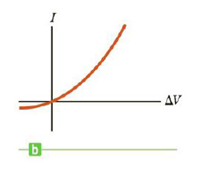Chapter 17.4, Problem 17.4QQ

Chapter
Section
Textbook Problem

In Figure 17.9b does the resistance of the diode (a) increase or (b) decrease as the positive voltage Δ V increases?To determine
Whether the resistance of diode is increasing or decreasing.

Explanation

Given Info:

Explanation:

From the graph the resistance can be founds R=ΔV/ΔI

R is the resistance of the diode, ΔV is the potential difference, and ΔI the current.

Conclusion: From figure 17

Still sussing out bartleby?

Check out a sample textbook solution.

See a sample solution

The Solution to Your Study Problems

Bartleby provides explanations to thousands of textbook problems written by our experts, many with advanced degrees!

Get Started

Find more solutions based on key concepts